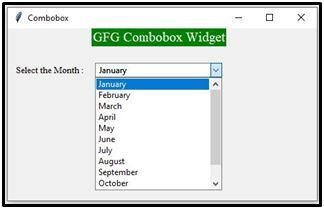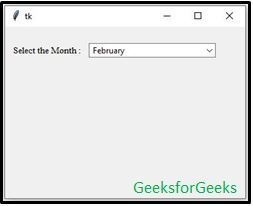# Combobox Widget in tkinter | Python

Python provides a variety of GUI (Graphic User Interface) types such as PyQT, Tkinter, Kivy, WxPython, and PySide. Among them, `tkinter` is the most commonly used GUI module in Python since it is simple and easy to understand. The word Tkinter comes from the Tk interface. The tkinter module is available in Python standard library which has to be imported while writing a program in Python to generate a GUI.

Note: Tkinter(capital T) is different from the tkinter. Tkinter is used in Python2.x and is changed to tkinter in Python.3x.

Combobox is a combination of Listbox and an entry field. It is one of the Tkinter widgets where it contains a down arrow to select from a list of options. It helps the users to select according to the list of options displayed. When the user clicks on the drop-down arrow on the entry field, a pop up of the scrolled Listbox is displayed down the entry field. The selected option will be displayed in the entry field only when an option from the Listbox is selected.

Syntax:

```combobox = ttk.Combobox(master, option=value, ...)
```

Example 1: Combobox widget without setting a default value.

 `# python program demonstrating ` `# Combobox widget using tkinter ` ` `  ` `  `import` `tkinter as tk ` `from` `tkinter ``import` `ttk ` ` `  `# Creating tkinter window ` `window ``=` `tk.Tk() ` `window.title(``'Combobox'``) ` `window.geometry(``'500x250'``) ` ` `  `# label text for title ` `ttk.Label(window, text ``=` `"GFG Combobox Widget"``,  ` `          ``background ``=` `'green'``, foreground ``=``"white"``,  ` `          ``font ``=` `(``"Times New Roman"``, ``15``)).grid(row ``=` `0``, column ``=` `1``) ` ` `  `# label ` `ttk.Label(window, text ``=` `"Select the Month :"``, ` `          ``font ``=` `(``"Times New Roman"``, ``10``)).grid(column ``=` `0``, ` `          ``row ``=` `5``, padx ``=` `10``, pady ``=` `25``) ` ` `  `# Combobox creation ` `n ``=` `tk.StringVar() ` `monthchoosen ``=` `ttk.Combobox(window, width ``=` `27``, textvariable ``=` `n) ` ` `  `# Adding combobox drop down list ` `monthchoosen[``'values'``] ``=` `(``' January'``,  ` `                          ``' February'``, ` `                          ``' March'``, ` `                          ``' April'``, ` `                          ``' May'``, ` `                          ``' June'``, ` `                          ``' July'``, ` `                          ``' August'``, ` `                          ``' September'``, ` `                          ``' October'``, ` `                          ``' November'``, ` `                          ``' December'``) ` ` `  `monthchoosen.grid(column ``=` `1``, row ``=` `5``) ` `monthchoosen.current() ` `window.mainloop() `

Output:Example 2: Combobox with initial default values.
We can also set the initial default values in the Combobox widget as shown in the below sample code.

 `import` `tkinter as tk ` `from` `tkinter ``import` `ttk ` ` `  `# Creating tkinter window ` `window ``=` `tk.Tk() ` `window.geometry(``'350x250'``) ` `# Label ` `ttk.Label(window, text ``=` `"Select the Month :"``,  ` `        ``font ``=` `(``"Times New Roman"``, ``10``)).grid(column ``=` `0``,  ` `        ``row ``=` `15``, padx ``=` `10``, pady ``=` `25``) ` ` `  `n ``=` `tk.StringVar() ` `monthchoosen ``=` `ttk.Combobox(window, width ``=` `27``,  ` `                            ``textvariable ``=` `n) ` ` `  `# Adding combobox drop down list ` `monthchoosen[``'values'``] ``=` `(``' January'``,  ` `                          ``' February'``, ` `                          ``' March'``, ` `                          ``' April'``, ` `                          ``' May'``, ` `                          ``' June'``,  ` `                          ``' July'``,  ` `                          ``' August'``,  ` `                          ``' September'``,  ` `                          ``' October'``,  ` `                          ``' November'``,  ` `                          ``' December'``) ` ` `  `monthchoosen.grid(column ``=` `1``, row ``=` `15``) ` ` `  `# Shows february as a default value ` `monthchoosen.current(``1``)  ` `window.mainloop() `

Output:My Personal Notes arrow_drop_upCheck out this Author's contributed articles.

If you like GeeksforGeeks and would like to contribute, you can also write an article using contribute.geeksforgeeks.org or mail your article to contribute@geeksforgeeks.org. See your article appearing on the GeeksforGeeks main page and help other Geeks.

Please Improve this article if you find anything incorrect by clicking on the "Improve Article" button below.

Article Tags :

2

Please write to us at contribute@geeksforgeeks.org to report any issue with the above content.